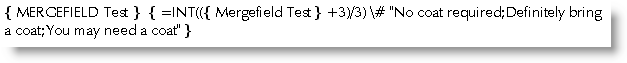# Graham Mayor

### ... helping to ease the lives of Microsoft Word users.## Negative numbers in Word calculations

Word does nothandle negative numbers correctly in calculated fields, because Word apparently makes the comparison between such items as alpha-numeric character groups rather than negative numbers.

Consider the circumstances where you have a database containing temperatures between 10° and -20° and you wish to present information in a mail merge based upon that data. For example, you may wish to recommend the use of a coat when the temperature is below -5° (the figure being chosen to demonstrate the problem rather than a climatic dress recommendation).Logic would suggest a simple conditional field as shown below:Running the merge produces the odd results listed below, which are clearly not what is required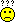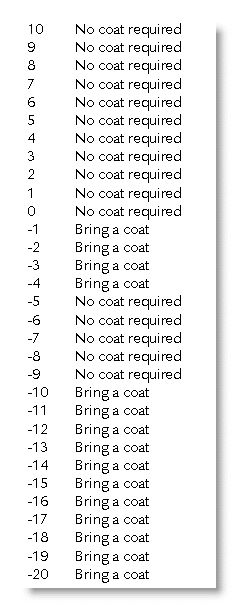One answer is to ensure that calculations are only conducted with positive numbers, to which end the ABS function will come in useful. Thus to modify the previous example, we first separate all the negative items (items less than 0) from the positive items, then deal with each separately, using nested IF fields as shown in the following example.

The first condition produces the result "No coat required" for all positive temperatures (those of 0° or above).

Negative numbers are converted to positive, then further compared to establish whether they are greater than 5. As the numbers are now positive, numbers greater than 5 are the numbers that were formerly below -5°.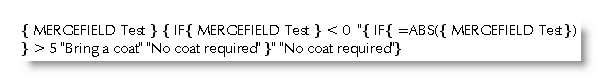Alternatively, you could use the SIGN function to establish whether or not numbers are negative. The effect is the same.Even simpler you could use the IF function, which can be used to return 1 or 0 and which can be used with a conditional field to produce the required result: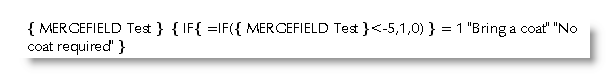#### Some alternative approaches

Instead of comparing against the negative number directly, simply add the corresponding positive value to the mergefield, then compare the result against 0Re-running the merge with any of the above produces the following, now correct, result: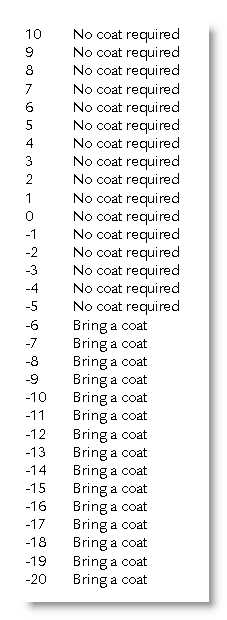Do away with the IF test altogether. Instead, use a formula field to add the corresponding positive value to the mergefield, then format the result with a picture switch. Note the third option in the switch. The result of this is shown at -5 in the listing below.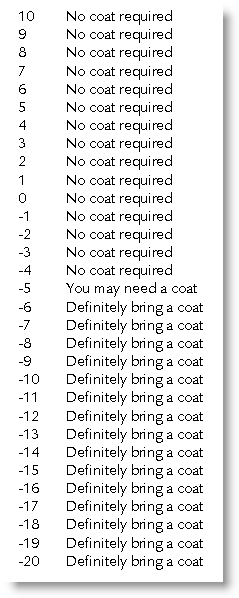The use of a picture switch with a little more mathematics allow the use of a range of values as shown below. The third option is produced when the result of the calculation is 0. By manipulation that calculation you can change the range.